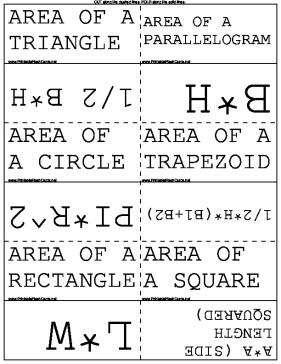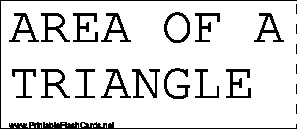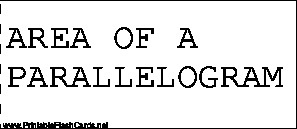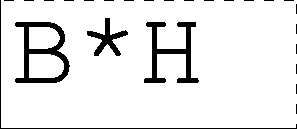Sample flash cards in this set:

Front:Back:This set includes the following cards:
AREA OF A TRIANGLE1/2 B*H
AREA OF A PARALLELOGRAMB*H
AREA OF A CIRCLEPI*R^2
AREA OF A TRAPEZOID1/2*H*(B1+B2)
AREA OF A RECTANGLEL*W
AREA OF A SQUAREA*A (SIDE LENGTH SQUARED)
PERIMETER OF TRIANGLEADD UP ALL THE SIDES
PERIMETER OF PARALLELOGRAM2A+2B
PERIMETER OF A CIRCLE (CIRCUMFERENCE)2*PI*R
PERIMETER OF A TRAPEZOIDADD ALL SIDES UP
PERIMETER OF A RECTANGLE2L+2W
PERIMETER OF A SQUARE4S